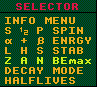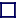### ZAN BEmax MODE INSTRUCTIONS

This plot (and the 'STABILITY' mode plot) plots the decay stabilities as calculated by the differences in atomic masses of neighbouring nuclides, and as such is different to the 'DECAY MODE' plot which displays the OBSERVED decay modes. Any discrepancies between the two are usually as a result of the branching ratio for one decay mode being so low that the decay is un-observed in practice. This applies especially to alpha instabilities where the mass difference is less than 2MeV; here the decays, although theoretically possible, is so improbable as to be unobservable in practice.

If Z BEmax is chosen, then the isotope having the maximum binding energy for each atomic number, Z, or element, are encircled in white. This plot nicely highlights the neutron numbers where N is magic.

If A BEmax is chosen, then the isobar having the maximum binding energy for each atomic weight, A, are encircled in white. This plot nicely highlights the isobars which would be stable to beta/inverse beta decay (all isobars would have at least one stable member if it weren't for alpha decay or spontaneous fission).

If N BEmax is chosen, then the isotone having the maximum binding energy for each neutron number, N, are encircled in white. This plot nicely highlights the atomic numbers where Z is magic.

To select these modes singly or in any combination, position the pointer over 'Z A N BEmax' and press any combination of the mouse buttons simultaneously. The left button represents the Z, the middle the A and the right button the N modes.